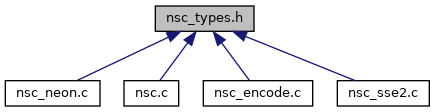FreeRDP
nsc_types.h File Reference
`#include <freerdp/config.h>`
`#include <winpr/crt.h>`
`#include <winpr/wlog.h>`
`#include <winpr/collections.h>`
`#include <freerdp/utils/profiler.h>`
`#include <freerdp/codec/nsc.h>`
This graph shows which files directly or indirectly include this file:## Data Structures

struct  NSC_CONTEXT_PRIV

struct  NSC_CONTEXT

## Macros

#define ROUND_UP_TO(_b, _n)   (_b + ((~(_b & (_n - 1)) + 0x1) & (_n - 1)))

#define MINMAX(_v, _l, _h)   ((_v) < (_l) ? (_l) : ((_v) > (_h) ? (_h) : (_v)))

## ◆ MINMAX

 #define MINMAX ( _v, _l, _h ) ((_v) < (_l) ? (_l) : ((_v) > (_h) ? (_h) : (_v)))

## ◆ ROUND_UP_TO

 #define ROUND_UP_TO ( _b, _n ) (_b + ((~(_b & (_n - 1)) + 0x1) & (_n - 1)))

FreeRDP: A Remote Desktop Protocol Implementation NSCodec Library

```http://www.apache.org/licenses/LICENSE-2.0